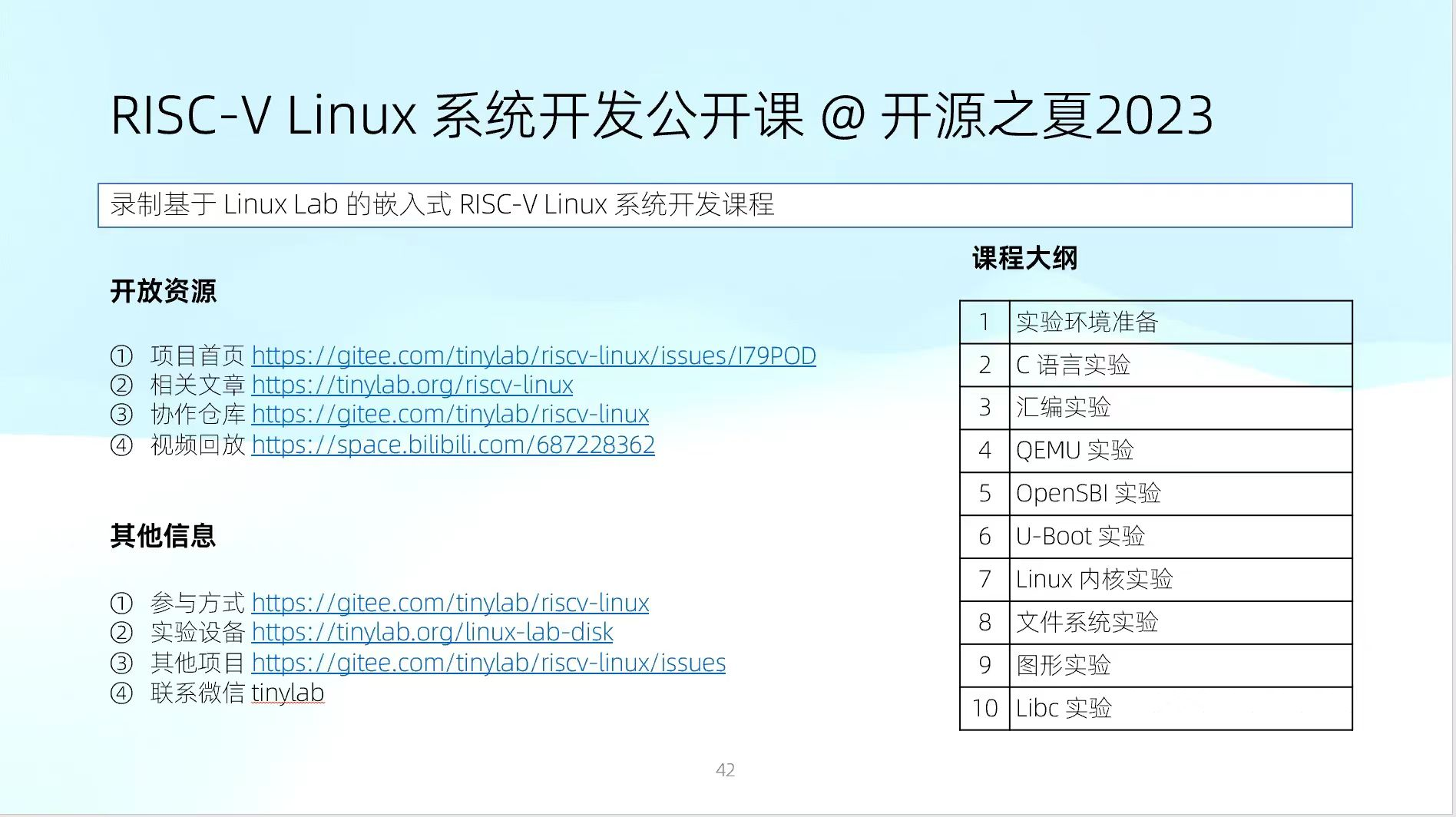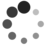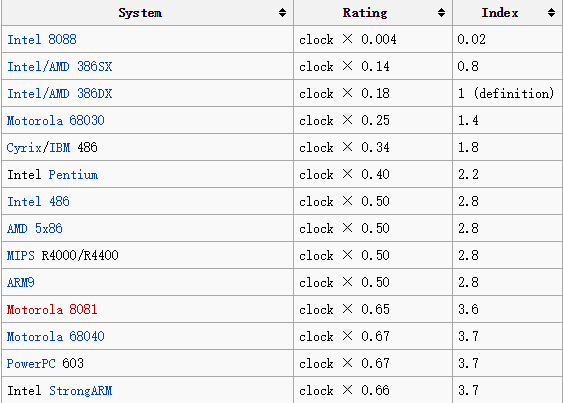# 内核探索：Linux BogoMips 探秘

By Tao Hongliang of TinyLab.org 2015/04/12

## BogoMIPS 的由来

BogoMIPS 是 Linus 本人的独创，Bogo 意思是“假的，伪造的”，MIPS 意思是“Millions of Instructions Per Second”，如果系统启动时，计算出 BogoMIPS 为 100，可记为 100万条伪指令每秒。

## BogoMIPS 的计算

```seq_printf(m, "BogoMIPS\t\t: %u.%02u\n",
cpu_data[n].udelay_val / (500000/HZ),
(cpu_data[n].udelay_val / (5000/HZ)) % 100);
```

```cpu_data[cpu].udelay_val = loops_per_jiffy;
```

```#define LPS_PREC 8

static unsigned long calibrate_delay_converge(void)
{
/* First stage - slowly accelerate to find initial bounds */
int trials = 0, band = 0, trial_in_band = 0;

lpj = (1<<12);

/* wait for "start of" clock tick */
/* 这里很聪明的选择了一个计算 loops 的起始时间，即，一个 tick 刚开始的时候 */
ticks = jiffies;
while (ticks == jiffies)
; /* nothing */
/* Go .. */
ticks = jiffies;

/* 这里用逐渐逼近的方式计算在一个jiffy的时间段内，循环调用 __delay（NOP 循环）,
* 最后累计 delay 了多少。loops_per_jiffy 就是多少了。
*/
do {
if (++trial_in_band == (1<<band)) {
++band;
trial_in_band = 0;
}
__delay(lpj * band);
trials += band;
} while (ticks == jiffies);
/*
* We overshot, so retreat to a clear underestimate. Then estimate
* the largest likely undershoot. This defines our chop bounds.
*/
trials -= band;
lpj_base = lpj * trials;

/* 接下来，再对上面算出来的 loops_per_jiffy 的值进行微调，确保其准确 */
recalibrate:
lpj = lpj_base;

/*
* Do a binary approximation to get lpj set to
* equal one clock (up to LPS_PREC bits)
*/
chop_limit = lpj >> LPS_PREC;
ticks = jiffies;
while (ticks == jiffies)
; /* nothing */
ticks = jiffies;
__delay(lpj);
if (jiffies != ticks)   /* longer than 1 tick */
}
/*
* If we incremented every single time possible, presume we've
* massively underestimated initially, and retry with a higher
* start, and larger range. (Only seen on x86_64, due to SMIs)
*/
if (lpj + loopadd * 2 == lpj_base + loopadd_base * 2) {
lpj_base = lpj;
goto recalibrate;
}

return lpj;
}
```

```BogoMIPS = loops_per_jiffy ÷ (500000 / HZ)   --->   BogoMIPS = (loops_per_jiffy * HZ) ÷ 500000
```

HZ 是什么，HZ 就是每秒的滴答数，即每秒的 jiffy 数。那么，loops_per_jiffy * HZ = loops_per_second

```BogoMIPS = loops_per_second ÷ 500000  --->   BogoMIPS = (loops_per_second * 2) ÷ 1000000
```

## BogoMIPS 和 CPU 频率的关系

• CPU 频率越快，loops_per_second 的值必然越大，那么 BogoMIPS 的值将会越大；
• CPU 频率越低，则 BogoMIPS 的值将越小；
• CPU 变频的时候，BogoMIPS 会随着 CPU 频率升高而升高，降低而降低。